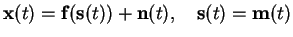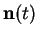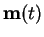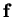Next: Learning algorithms Up: Nonlinear state-space models Previous: Multilayer perceptrons   Contents

## Nonlinear factor analysis

The NSSM implementation in  uses MLP networks to model the two nonlinear mappings in Equation (4.13). The learning procedure for the mappings is essentially the same as in the simpler NFA model , so it is presented first. The NFA model is also used in some of the experiments to explore the properties of the data set used.

Like the NSSM is a generalisation of the linear SSM, the NFA is a generalisation of the well-known linear factor analysis. The NFA can be defined with a generative data model given by(4.16)

where the noise termsandare assumed to be Gaussian. The explicit mention of the factors being modeled as white noise is included to emphasise the difference between the NFA and the NSSM. The Gaussianity assumptions are made because of mathematical convenience, even though the Gaussianity of the factors is a serious limitation.

In the corresponding linear model the posterior will be an uncorrelated Gaussian, and in the nonlinear model it is approximated with a similar Gaussian. However, an uncorrelated Gaussian with equal variances in each direction is spherically symmetric and thus invariant with respect to all possible rotations of the factors. Even if the variances are not equal, there is a similar rotation invariance.

In traditional approaches to linear factor analysis this invariance is resolved by fixing the rotation using different heuristic criteria in choosing the optimal one. In the neural computation field the research has lead to algorithms like independent component analysis (ICA)  which uses basically the same linear generative model but with non-Gaussian factors. Similar techniques can also be applied to nonlinear model as discussed in .

The NFA algorithm uses an MLP network as the model of the nonlinearity. The model is learnt using ensemble learning. Most of the expectations needed for ensemble learning for such a model can be evaluated analytically. Only the terms involving the nonlinearitymust be approximated by using Taylor series approximation for the function about the posterior mean of the input. The weights of the MLP network are updated with a back-propagation-like algorithm using the ensemble learning cost function. The unknown inputs of the network are also updated similarly, contrary to the standard supervised back-propagation.

All the parameters of the model are assumed to be Gaussian with hierarchical priors for most of them. The technical details of the model and the learning algorithm are covered in Chapters 5 and 6. Those chapters do, however, deal with the more general NSSM but the NFA emerges as a special case when all the temporal structure is ignored.Next: Learning algorithms Up: Nonlinear state-space models Previous: Multilayer perceptrons   Contents
Antti Honkela 2001-05-30